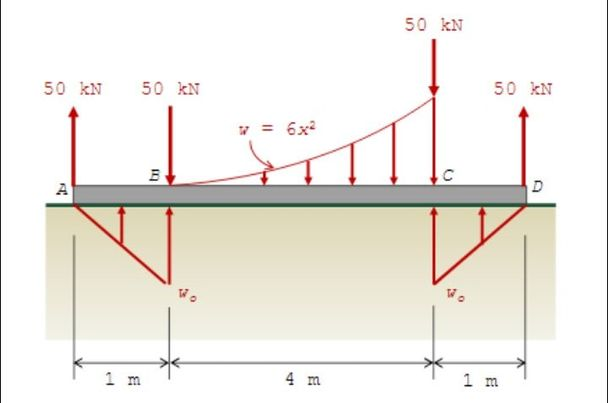# work problem

## shear and moment diagramA. determine Wo
B. Draw the shear and moment diagrams and specify the numerical values at all change of loading positions & at all points of zero shear.
C. determine the maximum shear and maximum moment.

## Mechanics of fluids

The flow rate of water through the turbine shown is 0.20 m^3/s and the pressure at points 1 and 2 are 150KPa and -35KPa. Determine the power generated by the turbine.

## solution for the question below

write the shear moment equations for the uniform beam below and draw the shear force and bending diagrams. specify values at all change of lading positions and at points of zero shear. the beam has a weight of 2kN

## Algebra

A 1,260-galloon tank is filled by two pipes in as many as the smaller pipe brings in galloons per minute. How long will it take each pipe to fill the tank if the larger pipe brings in one galloon per minute more than the smaller pipe?

## Algebra

A company has a certain number of machines of equal capacity that produced a total of 180 pieces each working day. If two machines breakdown, the workload of the remaining machines is increased by three pices per day to maintain production. Find the number of machines?## analysis of truss

any idea how to analyze trusses? like in method of sections and method of joint thanks in advance

## vector analysis

how to solve the tension force of a two ropes connected in a box of 100lb and is being hang. the angles of the ropes are 60? Meaninig the box is in the center.

## Solution to Problem 830

I'm learning how to do reactions with the Three Moment Theorem and in the solution for problem 830, it has 1077.27/12 = 538.64.....this clearly isn't correct.

Am I missing something? I tried searching to see if this has been addressed elsewhere, but some guidance would be very helpful and many thanks in advance.## Differential Equation

Guys may complete solution ba kayo sa mga problems sa DE..?## Finding the terminal points

The segment joining (1 -3) and (4 -6) is extended a distance equal to one sixth of its own length. find the terminal points.
How to solve this problem. I need the whole SOLUTION. THANKS

## Pages